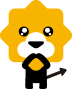• 骆驼(CAMEL)
• 木林森(MULINSEN)
• 红蜻蜓(REDDRAGONFLY)
• 奥康(AOKANG)
• 花花公子(PLAYBOY)
• 策乐(cele)
• 百丽(BeLLE)
• 英伦班纳(ENLEN&BENNA)
• 梦特娇(Montagut)
• 公牛世家(G.N.Shi Jia)
• 沙驰(SATCHI)
• 百思图(BASTO)
• 热风(hotwind)
• 羊骑士
• 富贵鸟(FUGUINIAO)
• Apple
• 铭爵帝皇(Ming Jue Di Huang)
• 森马(senma)
• 圆头
• 尖头
• 方头
• 扁头
• 鱼嘴
• 其他
• 反绒皮
• 头层牛皮
• 磨砂皮
• 羊皮
• 绒面
• 牛仔布
• 麂皮
• PU
• 绸缎
• 布面
• 毛线
• 塑胶
• 网面
• 牛二层皮
• 树脂
• 漆皮
• 太空革
• 超纤
• 猪皮
• 羊皮毛一体
• 混合材质
• 头层牛皮（除牛反绒）
• 网纱
• 网布
• 人造革
• 超纤皮
• 头层猪皮
• 二层猪皮
• 二层牛皮（除牛反绒）
• 牛反绒
• 棉布
• 无内里
• 袋鼠皮
• 纯羊毛
• 狐狸毛
• 仿毛
• 灯芯绒
• 涤沦
• 珍珠鱼皮
• 羊毛羊绒混纺
• 人造长毛绒
• 人造短毛绒
• 其他
• 羊反绒（羊猄）
• 鳄鱼皮
• 马皮
• 羊皮（除羊反绒/羊猄）
• 亮片布
• 腹膜皮
• 高丝光反绒皮
• 马毛
• 孔雀皮
• 塑胶
• 复合底
• 橡胶
• TPR(牛筋）
• 真皮
• 橡胶发泡
• 聚氨酯
• EVA发泡胶
• 泡沫
• EVA
• PVC
• PU
• 烟胶
• 软木
• MD
• 塑料
• tpu-

75944